# Vertical Throw Up

In the last lecture we learned about the motion of throwing an object down, now we want to investigate the motion of throwing an object up!

## Vertical Throw Up

An object thrown upward reaches the highest point and then turns around and falls, which is also a type of falling motion.

Throwing up seems difficult at first glance because the direction of motion changes during the process, but the important thing to remember is that the object is in motion only under gravity, both when it is moving upward and when it is falling downward.
This means that vertical throw up is also a uniform accelerated motion.

Check it out in the figure!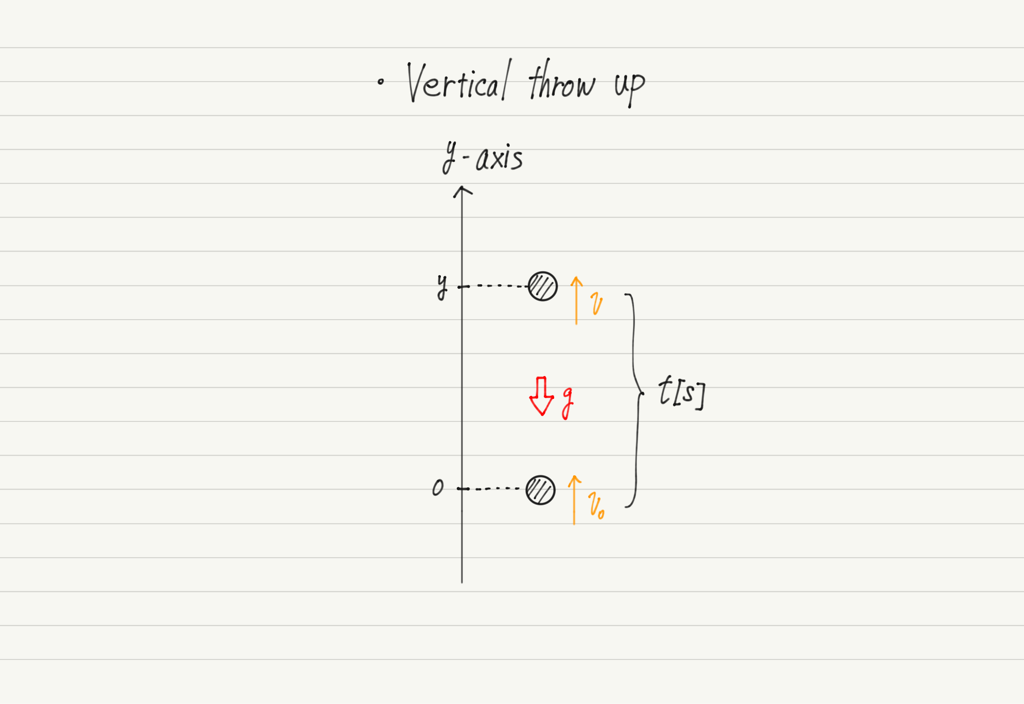Notice the orientation of the y-axis. In the case of the throw down, the downward direction was assumed to be positive, but this time the upward direction is assumed to be positive.

Since the upward direction is positive, gravitational acceleration occurs in a negative direction.

I am tempted to reconsider, “It would be troublesome to have a minus sign, so shouldn’t the axis be positive downward?”
But if you do so, a minus value is attached to the initial velocity.

In the end, no matter which direction is taken as positive, we cannot escape from the negative.
In such cases, the convention is to “take the direction of the initial velocity as positive”, so let’s just follow the convention.

To return to the topic, the vertical throw up motion is a uniform accelerated motion with “negative gravitational acceleration” as shown in the figure above.
Therefore, the formulas of vertical throw up is as follows.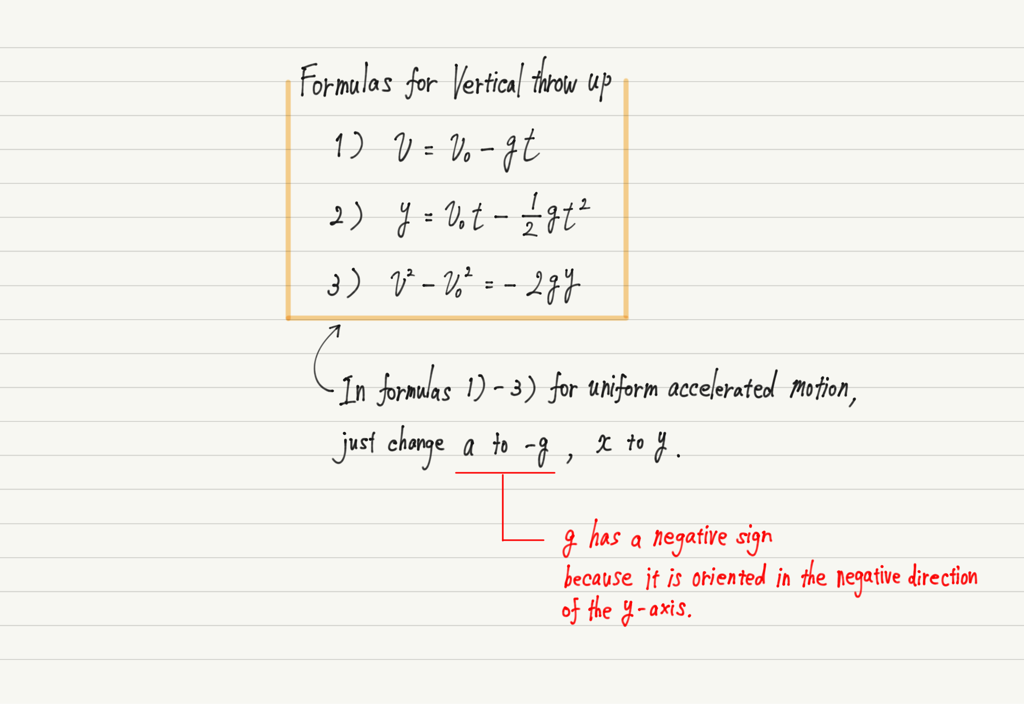As explained in the lecture on acceleration, the minus sign attached to acceleration can mean either “deceleration in the positive direction” or “acceleration in the negative direction.

https://www.yukimura-physics.com/entry/dyn-f05

In the case of a vertical throw up, it means “deceleration in the positive direction” from the throw up to the highest point, and “acceleration in the negative direction” after the highest point.
Note that during the throw-up motion, the acceleration is constant at –g throughout, but the meaning changes after the highest point.

## Graph of vertical throw up

Let’s also look at the graph of a vertical throw up. Since acceleration is negative, the v-t graph will look like the one below.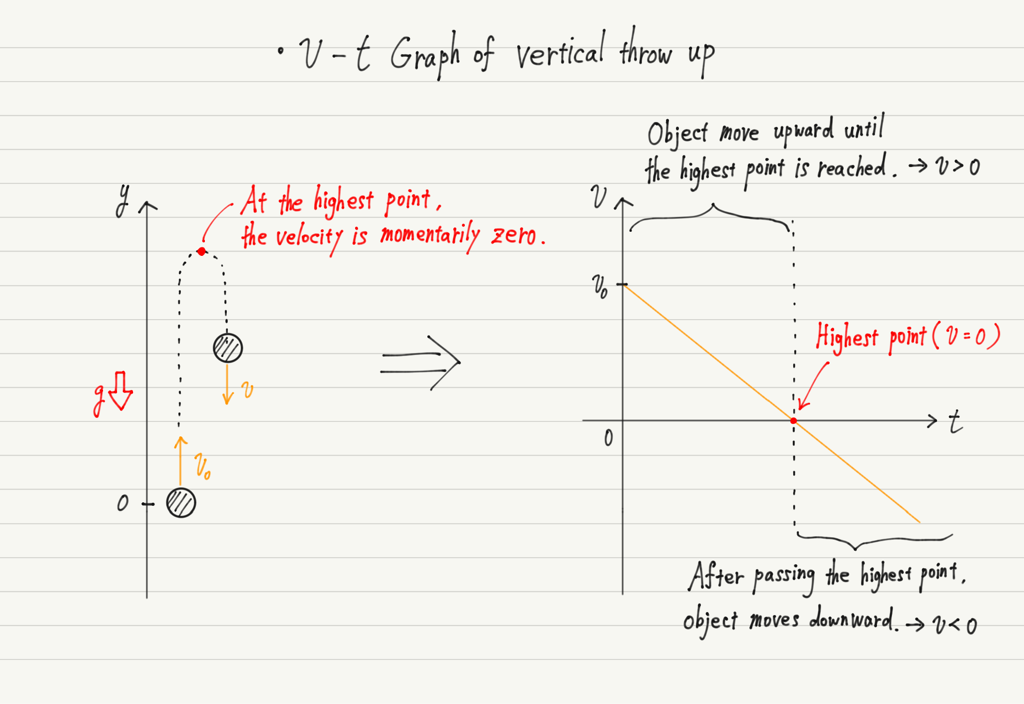The “Highest point = Zero velocity” is often used when solving problems.
Instead of memorizing it, please visualize the motion and be convinced that “At the highest point, the motion is stationary (only for a moment)”.

The y-t graph then follows from the throw up formula 2).This leads us to another important point.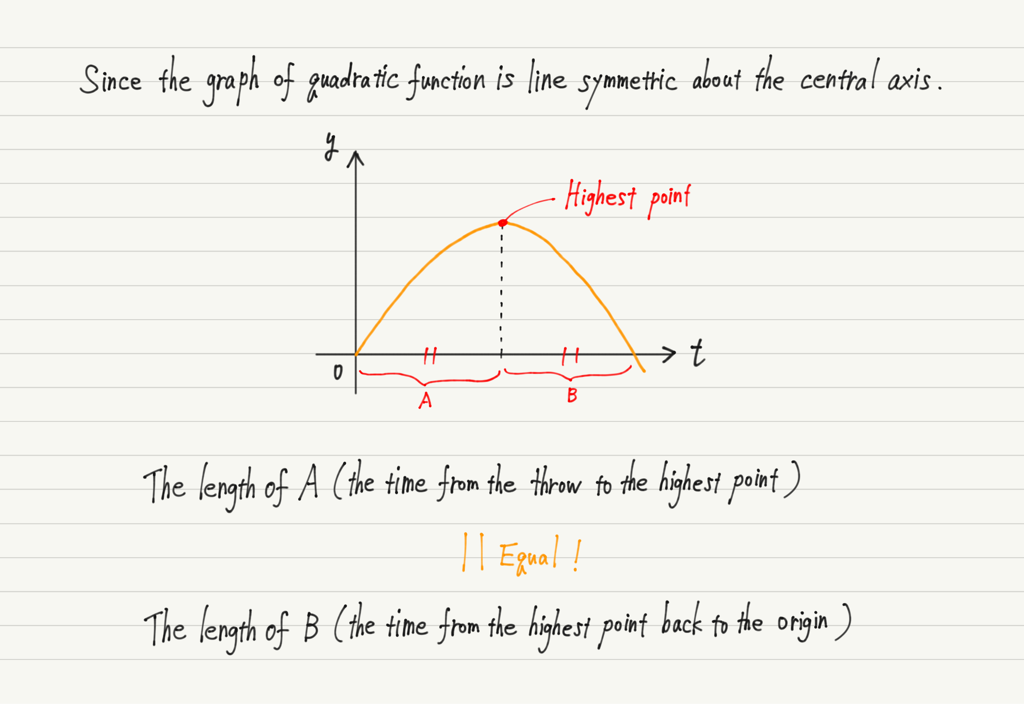Knowing the symmetry of this motion will be a big help when solving problems, so be sure to keep it in mind!

## Summary of this lecture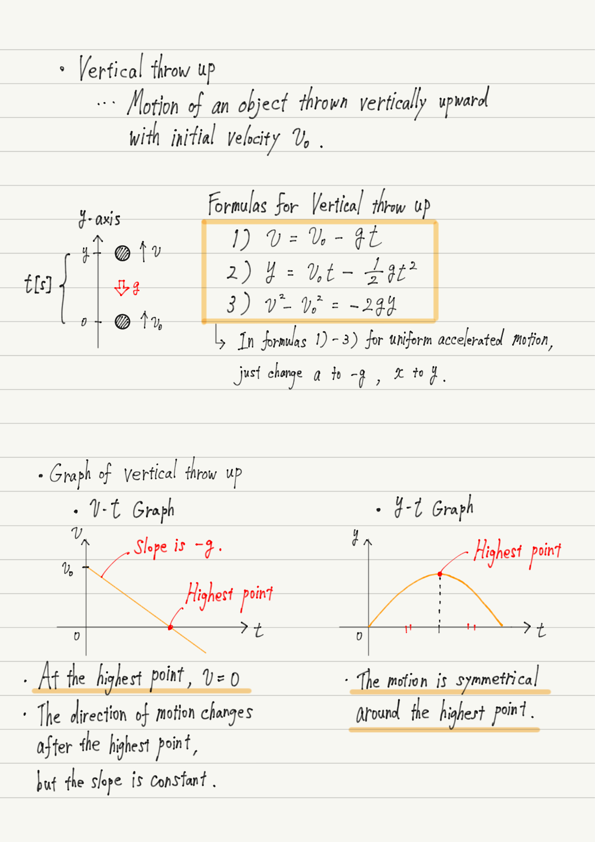## Next Time

From the next lecture, we will study forces, which are the key to mechanics.

https://www.yukimura-physics.com/entry/dyn-f10

error: Content is protected !!